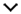You are here: Home » PF » Features » Others••# This is how your money grows...

## MFs: Where are the investors?

An insurance agent tries to sell a policy by pitching possible returns: “Invest Rs 20,000 as premium for ten years. At the end of tenure, you will get back Rs 3 lakh!”

A quick calculation seems to tell that you are investing Rs 2 lakh, and getting back Rs 3 lakh, a return of 50 per cent. In addition, you get an insurance cover too. This is incorrect!

The actual annual return is just 7 per cent because of money compounding year-on-year.

This is a concept called the time value of money. This concept deals with the idea that money available at the present time is worth more than the same amount in the future due to its potential earning capacity.

Therefore, the Rs 3 lakh in your hands today is much higher than Rs 3 lakh that you will receive in the future.

Let’s say you have invested Rs 1,000 in a 2-year fixed deposit that has an interest rate of 10 per cent. After a year, you have Rs 1,100 in the account, and Rs 1,210 (10 per cent of Rs1,100 added to Rs1,100) after two years. So, if you look at this Rs 1,210 in the future, it’s the same as today’s Rs 1,000. That’s of course, assuming a return of 10 per cent. In other words, Rs 1,210 is called the ‘future value’ of today’s Rs 1,000.

To calculate future value, we assume that today’s value (called the ‘present value’),earns interest on a compounded basis. The formula: Future Value = Present Value x (1+r/100)^t. The ‘r’ is the rate of return or interest (10 per cent in our example)and t is the time period (2 years). Go on, try it.

Let’s flip this calculation, and examine the Rs 3 lakh insurance agent promised ten years from now.

We also know that it’s the future value of a series of investments (Rs 20,000 each year), made over 10 years. Let us find out the value of this Rs 3 lakh today. We can use the same formula above. Here, we are aware of the future value and need to find out the present value. This process is called ‘discounting’.

To calculate the present value, we need to see two things. One, is the time period for which you need to discount it. In our example, that’s 10 years. The second, and most important, is the assumption of return – how much value or return do we assume it has accumulated? Let us assume 7 per cent. Arithmetically, present value = future value/(1+r)^t

The Present Value works out to: 3,00,000/1.07^5 = Rs 1,52,504.

It is less than the investment made, so does that mean negative returns? No, it doesn’t. We must find the present value of each of our premiums as well. The present value of the first Rs 20,000 is Rs 20,000 itself, as it is being paid today. The present value of the second Rs 20,000, a year from now will be: 20,000/1.07^1, and so on.

If we now add up all the present values, the result is Rs1,52,504 as well. What this simply means is that the present value of all your outflows (premiums) is equal to the present value of your inflow at a rate of return of 7 per cent.

This is the actual return on your investment and not 50 per cent, as we mentioned before.

Lastly, we assumed a return of 7 per cent, because I knew the answer. If you don’t, just presume a return and work around it to find out the rate at which both present values are the same. You can do that as an exercise, by taking your own insurance policy.

This concept of time value is used across the world of finance. You need it when you are saving for a goal like education, marriage or taking a loan to create an asset.

The author is CEO of www.learnwithflip.com

First Published: Sun, July 25 2010. 00:40 IST